Note: A new GRASS GIS stable version has been released: GRASS GIS 7.8, available here.
Updated manual page: here

## NAME

r.slope.aspect - Generates raster maps of slope, aspect, curvatures and partial derivatives from an elevation raster map.
Aspect is calculated counterclockwise from east.

## KEYWORDS

raster, terrain, aspect, slope, curvature

## SYNOPSIS

r.slope.aspect
r.slope.aspect --help
r.slope.aspect [-aen] elevation=name [slope=name] [aspect=name] [format=string] [precision=string] [pcurvature=name] [tcurvature=name] [dx=name] [dy=name] [dxx=name] [dyy=name] [dxy=name] [zscale=float] [min_slope=float] [--overwrite] [--help] [--verbose] [--quiet] [--ui]

### Flags:

-a
Do not align the current region to the raster elevation map
-e
Compute output at edges and near NULL values
-n
Create aspect as degrees clockwise from North (azimuth), with flat = -9999
Default: degrees counter-clockwise from East, with flat = 0
--overwrite
Allow output files to overwrite existing files
--help
Print usage summary
--verbose
Verbose module output
--quiet
Quiet module output
--ui
Force launching GUI dialog

### Parameters:

elevation=name [required]
Name of input elevation raster map
slope=name
Name for output slope raster map
aspect=name
Name for output aspect raster map
format=string
Format for reporting the slope
Options: degrees, percent
Default: degrees
precision=string
Type of output aspect and slope maps
Storage type for resultant raster map
Options: CELL, FCELL, DCELL
Default: FCELL
CELL: Integer
FCELL: Single precision floating point
DCELL: Double precision floating point
pcurvature=name
Name for output profile curvature raster map
tcurvature=name
Name for output tangential curvature raster map
dx=name
Name for output first order partial derivative dx (E-W slope) raster map
dy=name
Name for output first order partial derivative dy (N-S slope) raster map
dxx=name
Name for output second order partial derivative dxx raster map
dyy=name
Name for output second order partial derivative dyy raster map
dxy=name
Name for output second order partial derivative dxy raster map
zscale=float
Multiplicative factor to convert elevation units to horizontal units
Default: 1.0
min_slope=float
Minimum slope value (in percent) for which aspect is computed
Default: 0.0

## DESCRIPTION

r.slope.aspect generates raster maps of slope, aspect, curvatures and first and second order partial derivatives from a raster map of true elevation values. The user must specify the input elevation raster map and at least one output raster maps. The user can also specify the format for slope (degrees, percent; default=degrees), and the zscale: multiplicative factor to convert elevation units to horizontal units; (default 1.0).

The elevation input raster map specified by the user must contain true elevation values, not rescaled or categorized data. If the elevation values are in other units than in the horizontal units, they must be converted to horizontal units using the parameter zscale. In GRASS GIS 7, vertical units are not assumed to be meters any more. For example, if both your vertical and horizontal units are feet, parameter zscale must not be used.

The aspect output raster map indicates the direction that slopes are facing counterclockwise from East: 90 degrees is North, 180 is West, 270 is South, 360 is East. Zero aspect indicates flat areas with zero slope. Category and color table files are also generated for the aspect raster map.
Note: These values can be transformed to azimuth values (90 is East, 180 is South, 270 is West, 360 is North) using r.mapcalc:

```# convert angles from CCW from East to CW from North
# modulus (%) can not be used with floating point aspect values
r.mapcalc "azimuth_aspect = if(ccw_aspect == 0, 0, \
if(ccw_aspect < 90, 90 - ccw_aspect, \
450 - ccw_aspect)))"
```
Alternatively, the -n flag can be used to produce aspect as degrees CW from North. Aspect for flat areas is then set to -9999 (default: 0).

The aspect for slope equal to zero (flat areas) is set to zero (-9999 with -n flag). Thus, most cells with a very small slope end up having category 0, 45, ..., 360 in aspect output. It is possible to reduce the bias in these directions by filtering out the aspect in areas where the terrain is almost flat. A option min_slope can be used to specify the minimum slope for which aspect is computed. For all cells with slope < min_slope, both slope and aspect are set to zero.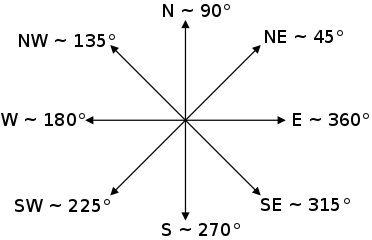The slope output raster map contains slope values, stated in degrees of inclination from the horizontal if format=degrees option (the default) is chosen, and in percent rise if format=percent option is chosen. Category and color table files are generated.

Profile and tangential curvatures are the curvatures in the direction of steepest slope and in the direction of the contour tangent respectively. The curvatures are expressed as 1/metres, e.g. a curvature of 0.05 corresponds to a radius of curvature of 20m. Convex form values are positive and concave form values are negative.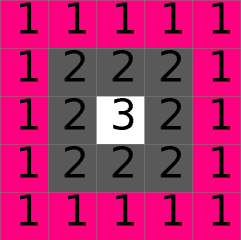Example DEM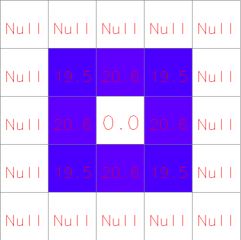Slope (degree) from example DEM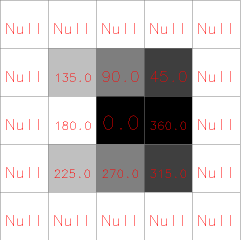Aspect (degree) from example DEM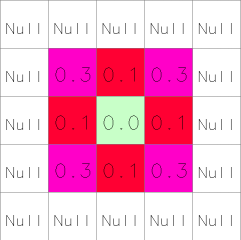Tangential curvature (m-1) from example DEM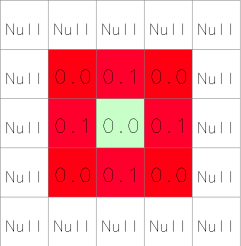Profile curvature (m-1) from example DEM

For some applications, the user will wish to use a reclassified raster map of slope that groups slope values into ranges of slope. This can be done using r.reclass. An example of a useful reclassification is given below:

```          category      range   category labels
(in degrees)    (in percent)

1         0-  1             0-  2%
2         2-  3             3-  5%
3         4-  5             6- 10%
4         6-  8            11- 15%
5         9- 11            16- 20%
6        12- 14            21- 25%
7        15- 90            26% and higher

The following color table works well with the above
reclassification.

category   red   green   blue

0       179    179     179
1         0    102       0
2         0    153       0
3       128    153       0
4       204    179       0
5       128     51      51
6       255      0       0
7         0      0       0```

## NOTES

To ensure that the raster elevation map is not inappropriately resampled, the settings for the current region are modified slightly (for the execution of the program only): the resolution is set to match the resolution of the elevation raster map and the edges of the region (i.e. the north, south, east and west) are shifted, if necessary, to line up along edges of the nearest cells in the elevation map. If the user really wants the raster elevation map resampled to the current region resolution, the -a flag should be specified.

The algorithm used to determine slope and aspect uses a 3x3 neighborhood around each cell in the raster elevation map. Thus, slope and aspect are not determineed for cells adjacent to the edges and NULL cells in the elevation map layer. These cells are by default set to nodata in output raster maps. With the -e flag, output values are estimated for these cells, avoiding cropping along the edges.

Horn's formula is used to find the first order derivatives in x and y directions.

Only when using integer elevation models, the aspect is biased in 0, 45, 90, 180, 225, 270, 315, and 360 directions; i.e., the distribution of aspect categories is very uneven, with peaks at 0, 45,..., 360 categories. When working with floating point elevation models, no such aspect bias occurs.

## EXAMPLES

### Calculation of slope, aspect, profile and tangential curvature

In this example a slope, aspect, profile and tangential curvature map are computed from an elevation raster map (North Carolina sample dataset):
```g.region raster=elevation
r.slope.aspect elevation=elevation slope=slope aspect=aspect pcurvature=pcurv tcurvature=tcurv

# set nice color tables for output raster maps
r.colors -n map=slope color=sepia
r.colors map=aspect color=aspectcolr
r.colors map=pcurv color=curvature
r.colors map=tcurv color=curvature
```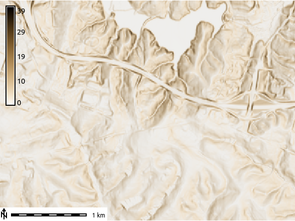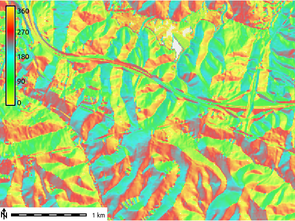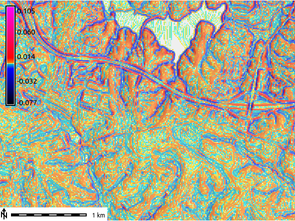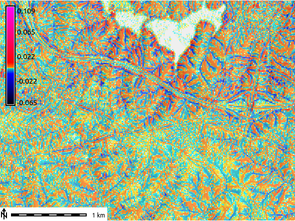Figure: Slope, aspect, profile and tangential curvature raster map (North Carolina dataset)

### Classification of major aspect directions in compass orientation

In the following example (based on the North Carolina sample dataset) we first generate the standard aspect map (oriented CCW from East), then convert it to compass orientation, and finally classify four major aspect directions (N, E, S, W):
```g.region raster=elevation -p

# generate integer aspect map with degrees CCW from East
r.slope.aspect elevation=elevation aspect=myaspect precision=CELL

# generate compass orientation and classify four major directions (N, E, S, W)
r.mapcalc "aspect_4_directions = eval( \\
compass=(450 - myaspect ) % 360, \\
if(compass >=0. && compass < 45., 1)  \\
+ if(compass >=45. && compass < 135., 2) \\
+ if(compass >=135. && compass < 225., 3) \\
+ if(compass >=225. && compass < 315., 4) \\
+ if(compass >=315., 1) \\
)"

# assign text labels
r.category aspect_4_directions separator=comma rules=- << EOF
1,north
2,east
3,south
4,west
EOF

# assign color table
r.colors aspect_4_directions rules=- << EOF
1 253,184,99
2 178,171,210
3 230,97,1
4 94,60,153
EOF
```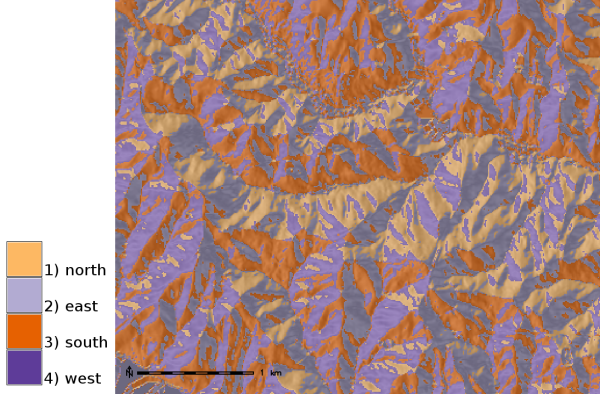Aspect map classified to four major compass directions (zoomed subset shown)

## REFERENCES

• Horn, B. K. P. (1981). Hill Shading and the Reflectance Map, Proceedings of the IEEE, 69(1):14-47.
• Mitasova, H. (1985). Cartographic aspects of computer surface modeling. PhD thesis. Slovak Technical University , Bratislava
• Hofierka, J., Mitasova, H., Neteler, M., 2009. Geomorphometry in GRASS GIS. In: Hengl, T. and Reuter, H.I. (Eds), Geomorphometry: Concepts, Software, Applications. Developments in Soil Science, vol. 33, Elsevier, 387-410 pp, http://www.geomorphometry.org

r.mapcalc, r.neighbors, r.reclass, r.rescale

## AUTHORS

Michael Shapiro, U.S.Army Construction Engineering Research Laboratory
Olga Waupotitsch, U.S.Army Construction Engineering Research Laboratory

Last changed: \$Date\$

## SOURCE CODE

Available at: r.slope.aspect source code (history)

Note: A new GRASS GIS stable version has been released: GRASS GIS 7.8, available here.
Updated manual page: here

© 2003-2020 GRASS Development Team, GRASS GIS 7.6.2dev Reference Manual